Courses

Test: Statistics- 2

20 Questions MCQ Test NCERT Mathematics for CAT Preparation | Test: Statistics- 2

Description
Attempt Test: Statistics- 2 | 20 questions in 40 minutes | Mock test for GMAT preparation | Free important questions MCQ to study NCERT Mathematics for CAT Preparation for GMAT Exam | Download free PDF with solutions
QUESTION: 1

The numbers in set P denote the distance of certain positive integers from -1 on the number line. The numbers in set Q denote the distance of the same integers from 1 on the number line. Which of the following statements is true about the standard deviation of the sets P and Q?

Solution:

Given:

• Let the integers be a, b, c, d, e….
• Set P = {Respective Distance of a, b, c, , d, e…. from -1}
• Set Q = {Respective Distance of a, b, c, d, e…from 1}

To Find: the statement, which is true about the standard deviation of sets P and Q

Approach:

1. To find the relation between the standard deviation of sets P and Q, we would need to find the relation between the terms that are present in sets P and Q
2. Terms in set P
• We know that distance of a number x from -1 on the number line can be written as |x –(-1)| = |x +1|
• We will use the above understanding to write down the terms of set P
3. Terms in set Q
• We know that distance of a number x from 1 on the number line can be written as |x -1|
• We will use the above understanding to write down the terms of set Q
4. Once we write down the terms of sets P and Q, we will find the relation between the terms of these 2 sets. Then, we'll use the standard properties of standard deviation to compare the standard deviation of sets P and Q.

Working out:

1. Terms in set P
• Set P = {|a+1|, |b+1|, |c+1|…….}
2. Terms in set Q
• Set Q = {|a-1|, |b-1|, |c-1|…….} = {|(a + 1) -2|, |(b+1) -2|, |(c+1) -2|……..}
• We can observe that if we subtract 2 from each of the terms of set P, we will get the terms of set Q.
3. We know from the property of standard deviation that reducing all the terms of a set by the same constant does not change the standard deviation of the set.
4. So, we can write Standard Deviation(P) = Standard Deviation(Q)

QUESTION: 2

List A: 20, 4, 8, x List B: 8, 3, 6, 12, -4 List A above has 4 numbers and List B above has 5 numbers. What is the value of x? (1) The range of the numbers in List A is equal to the range of the numbers in List B (2) The median of the numbers in List A is equal to the median of the numbers in List B.

Solution:

Step 1 & 2: Understand Question and Draw Inference

Given:

• List A: 4, 8, 20, x (don’t know the value of x, other numbers arranged in ascending order)
• List B: -4, 3, 6, 8, 12 (numbers arranged in ascending order)

To find: x = ?

Step 3 : Analyze Statement 1 independent

(1) The range of the numbers in List A is equal to the range of the numbers in List B

• Range of List B = 12 – (-4 ) = 12 + 4 = 16
• So, Range of List A = 16
• (Greatest number of List A) – (Smallest number of List A) = 16
• In List A, difference between 20 and 4 is 16
• This means, Greatest number of List A = 20
• And, Smallest number of List A = 4
• This means, 4 ≤ x ≤ 20

Not sufficient to find a unique value of x.

Step 4 : Analyze Statement 2 independent

(2) The median of the numbers in List A is equal to the median of the numbers
in List B.

• Median of List B = 6
• So, Median of List A = 6
• In List A, possible arrangements of the 4 elements in ascending order and the corresponding medians: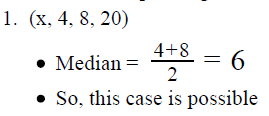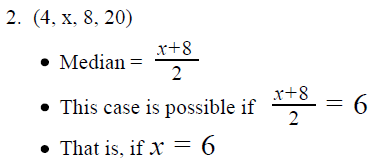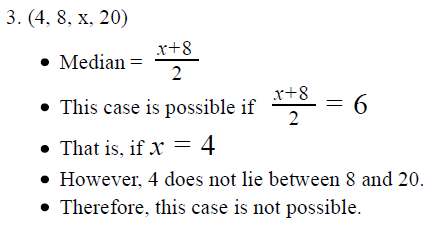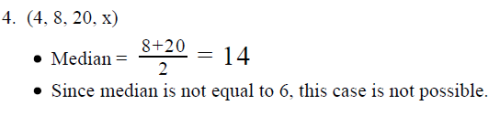• Thus, from Statement 2, we infer that x ≤ 4

Not sufficient to find a unique value of x

Step 5: Analyze Both Statements Together (if needed)

• From Statement 1: 4 ≤ x ≤ 20
• From Statement 2: x ≤ 4
• combing the 2 statements: x = 4Combin

Thus, the two statements together are sufficient to find a unique value of x

QUESTION: 3

When positive integer y is added to each of the first n non-negative integers, which of the following statements is true? I. If the median of the resulting numbers is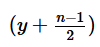then n is odd II. The arithmetic mean of the resulting numbers is equal to the median of the resulting numbers III. The arithmetic mean of the resulting numbers is y units greater than the arithmetic mean of the first n positive integers.

Solution:

Given:

• The list of first n non-negative integers: {0, 1, 2, 3, . . . , n – 1}
• Positive integer y is added to each integer in this list: {0 + y, 1 + y, 2 + y, . . . n – 1 + y}
• = {y, y + 1, y + 2, . . . , y + n – 1}

To Find: Which of the 3 statements is/are true?

Approach:

1. Since these 3 statements deal with:
• Mean of the first n positive integers
• Mean of the resulting numbers
• And, Median of the resulting numbers,

We will first find the expressions for these 3 quantities.

2. Then, we’ll evaluate the 3 statements one by one to determine which is/are true for all values of y and n

Working out:

• Finding the expressions for the 3 quantities featured in Statements I – III

• Finding Mean of the first n positive integers
• Sum of first n positive integers =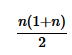• So, the mean of the first n positive integers =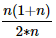• (n+2/2)
• Finding Mean of the Resulting Numbers
• The resulting numbers are: {y, y + 1, y + 2, . . . , y + n – 1}
• These numbers form an increasing arithmetic sequence of n terms.
• First term of the sequence = y
• Last term of the sequence = y + n -1
• So, the sum of these numbers =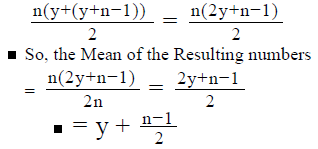• Finding Median of the Resulting Numbers
• The resulting numbers are: {y, y + 1, y + 2, . . . , y + n – 1}
• The total number of elements in this set is (y + n – 1) – y + 1 = n
• These numbers form an increasing arithmetic sequence of n terms.
• Now, in an ordered list that has:
• An even number of elements (say 4 elements), the median of the list is equal to the average of the middle 2 elements of the list
• An odd number of elements (Say 5 elements), the median of the list is equal to the middle element in the list

• Case 1: If n is odd,
• Then, Median = the middle element in the list of resulting numbers
• The first term in the list is y + 0 and the last term is y +(n – 1)
• So, the Median =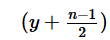• (Note: If the above expression for the Median is not intuitive to you, you can arrive at it by taking a few easy values of n. For example:
• If n = 3, the list is {y, y + 1, y + 2}. So, the median = y + 1
• If n = 5, the list is {y, y + 1. y + 2, y + 3, y + 4}. So, the median = y + 2
• Similarly, if n = 7, the list goes from y to y + 6 and the median = y + 3
• From these examples, the pattern for how the value of Median changes with n becomes easy to see)
• Case 2: If n is even,
• This means, the median of the list is equal to the​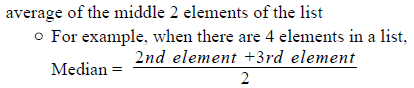•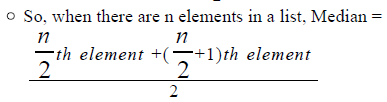•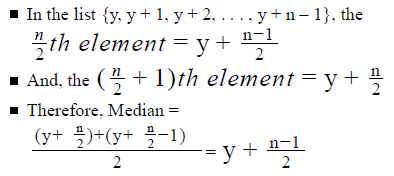• Evaluating Statement I
• If the median of the resulting numbers is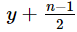then n is odd
• In our calculation of the Median of the Resulting Numbers, observe that the median is always equal to, whether n is even or odd.
• Therefore, Statement I is not correct
• Evaluating Statement II
• The arithmetic mean of the resulting numbers is equal to the median of the resulting numbers
• From our calculations of the Mean and Median of the Resulting Numbers, we see that:
• Mean of the Resulting numbers =• Median =• So, Statement II is indeed true.
• Evaluating Statement III
• The arithmetic mean of the resulting numbers is y units greater than the arithmetic mean of the first n positive integers.
• From our calculations above, we see that:
• Mean of the Resulting numbers =• Mean of the first n positive integers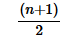• Note that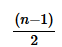is not equal to. Therefore, it is wrong to say that Mean of the Resulting Numbers is y units greater than the Mean of the first n positive integers.
• So, Statement III is not true.

• Of the 3 statements, we see that only Statement II is true.

Looking at the answer choices, we see that the correct answer is Option B

QUESTION: 4

If a, b and c are positive integers, what is the average (arithmetic mean) of a and c?
1. The average of a + b and 4 is 6
2. The average of a + c and b is 18

Solution:

Step 1 & 2: Understand Question and Draw Inference

Given: Integers a, b, c > 0
To find: (a+c) /2

Step 3 : Analyze Statement 1 independent

Statement 1 says that ‘The average of a + b and 4 is 6’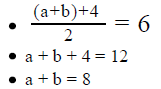• This equation doesn’t indicate to us the value of a + c
• So, Statement 1 is not sufficient to answer the question

Step 4 : Analyze Statement 2 independent

Statement 2 says that ‘The average of a + c and b is 18’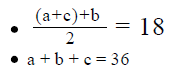• We need to find the value of a + c. However, the above equation gives us the value of a + b + c
• Multiple values of a + c will satisfy this equation
• For example, a + c = 35 and b = 1 or a + c = 34 and b = 2 etc.
• Therefore, Statement 2 alone is not sufficient to answer the question

Step 5: Analyze Both Statements Together (if needed)

• From Statement 1: a + b = 8
• From Statement 2: a + b + c = 36
• Combining the 2 statements: c = 36 – 8 = 28
• However, we do not yet know the value of a
• So, we are still unable to find the value of a + c
So, even the 2 statements together are not sufficient to answer the question

QUESTION: 5

In an inter-state Mathematics Olympiad, the distribution of the scores obtained by the participating students is symmetric about
the mean m. 68 percent of the distribution lies within one standard deviation d of the mean and 95 percent of the distribution lies
within 2 standard deviations of the mean. If there were 70 students who scored more than Ricky, 428 students who scored less than Ricky and none that scored equal to him, his score must lie between

Solution:

Given:

• 70 students scored more than Ricky
• 428 students scored less than Ricky
• None scored equal to Ricky
• Number of students = 70 + 428 + 1 = 499
• When arranged in ascending order, Ricky’s score would be at 429 place.
• Mean score = m
• Standard deviation of the scores = d

To Find:: The range in which Ricky’s score should lie

Approach:

1. As the options are given in terms of m and d, we first need to understand the distribution curve.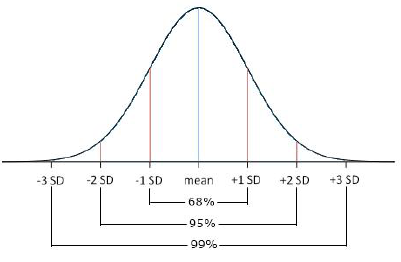2. The above distribution is symmetric about the mean m
• 68% of the distribution lies within 1 standard deviation, the distribution would have   68% / 2 = 34  of data sets on either sides of the mean.
• Similarly, 95% of the distribution lies within 2 standard deviation, the distribution would have 95 % / 2 = 47.5 % of data sets on either sides of the mean.
3. Since we know the position of Ricky’s score and we know the number of students who appeared in the Olympiad, we can calculate the number of students between consecutive standard deviations of the mean score.

Working out:

1. Since, 499 students appeared in the Olympiad, the mean score would lie at position 250
• So, number of students whose score lies within 1 standard deviation = 68% of 500 = 340
• So, number of students whose score is between m and m+d = 340 / 2 = 170  i.e. position of scores of students who lie between m and m + d will be between 250 and (250+170) = 420
• We need not bother with scores of students whose position is less than 250, as we are concerned about the position of student who is at 429th place.
2. As Ricky’s score is at 429 place, his score does not lie between m and m+ d. Let’s see if he lies between m + d and m + 2d.
3. Number of students whose score lie within 2 standard deviations = 95% of 500 = 475
• Number of students whose score lie between m and m + 2d = 475/2 = 237
4. So, number of students whose score lie between m + d and m + 2d = 237– 170 = 67
5. So, the position of students whose score lie between m + d and m + 2d will be between 420 and 420 + 67 = 487
• As Ricky’s score lies at a position of 429, his score would lie between m + d and m + 2d

QUESTION: 6

The average (arithmetic mean) of the prime numbers that lie between 10 and 20 is how much greater than the average of the
prime numbers that lie between 1 and 10?

Solution:

Given:

2 sets of Prime Numbers – let’s call them Sets A and B:

• Set A = {2, 3, 5, 7}
• Set B = {11, 13, 17, 19}

To find: Difference between Average(Set B) and Average (Set A)

• That is, Average(Set B) – Average (Set A)

Approach:

1. In order to find the answer, we need to know the values of Average(Set A), Average(Set B)
2. Since we know all the elements in each of Set A and B, we will be easily able to find the required averages.

Working out: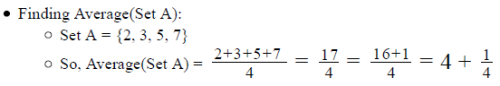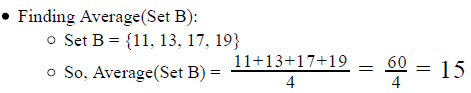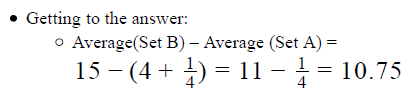QUESTION: 7

A group of students was participating in a quizzing competition consisting of 3 rounds. A student had to clear the first round to move into the second round and so on till he cleared all the rounds. What was the median number of the rounds cleared by the students in the quiz?
(1) 20 percent of the students could not clear round 1 of the quiz
(2) 40 percent of the students could clear round 2 but could not clear round 3.

Solution:

Step 1 & 2: Understand Question and Draw Inference

• Let the number of students be x
• Number of rounds in the quiz = 3

To Find: Median number of rounds cleared by the students

• Median = {0, 1, 2 or 3}

Step 3 : Analyze Statement 1 independent

1. 20  percent of the students could not clear round 1 of the quiz.
• 0.2x could not clear round 1
• 0.8x cleared round 1
• Since more than half cleared the first round, we can be sure that Median is not equal to zero
• But we do not know how many of them cleared round 2 and round 3.So,
• Median may be 1, 2 or 3

Step 4 : Analyze Statement 2 independent

2. 40 percent of the students could clear round 2 but could not clear round 3.

• 0.4x could clear round 2 but not clear round 3
• All the students who cleared Round 2 must have cleared Round 1 first
• This means, we can be sure that at least 0.4x students definitely cleared Round 1

However, we do not know the number of students who cleared round 3 and the number of students who could not clear round 1

Step 5: Analyze Both Statements Together (if needed)

1. From Statement 1, we inferred that 0.8x cleared round 1 and 0.2x didn’t
2. From Statement 2, we know that 0.4x could clear round 2 but not clear round 3

Let there be a% of students who cleared round 1 and b% of students who cleared round 2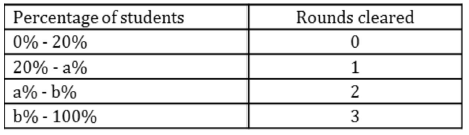• a% ≥ 20%
• b% ≤ 100%
• From st-2 we know that b% - a% = 40%. Since we do not know the division of the rest 40% of the students, we will not have a unique value for the median. For example:
• If a% = 20% and b% = 60%, median = 2
• If a% = 60% and y% = 100%, median = 1

QUESTION: 8

Arrange the following sets in the order of the increasing magnitude of their mean to standard deviation ratio.
I. {50, 60, 70, 80}
II. {35, 40, 45, 50}
III. {90, 110, 130, 150}

Solution:

Given:

• {50, 60, 70, 80}
• {35, 40, 45, 50}
• {90, 110, 130, 150}

To Find: Arrange the sets in increasing order of the (Mean / Standard deviation) ratio.

Approach:

1. Let’s assume the mean of set-I be m and standard deviation be d.
2. We would try to express the terms of the other two sets in terms of set-I for establishing a relation between the standard deviations and the means of the set
3. Also, we will use the following properties of standard deviation to calculate the standard deviation of the other sets in terms of d:
• If all the numbers of a set are multiplied or divided by the same constant x, the standard deviation is also multiplied or divided by |x| respectively.
• If all the numbers of a set are increased or decreased by the same constant x, the standard deviation of the set does not change.

Working out:

1. Set-I: {50, 60, 70, 80}
• Assuming the mean of set {50, 60, 70, 80} as m and standard deviation be d
• Ratio = m/d………(1)
2. Set-II: {35, 40, 45, 50}
• {50, 60, 70, 80} → Dividing all the terms of the set by 2, results in the standard deviation of d/2 and mean of m/2 . The set becomes = {25, 30, 35, 40}
• Adding 10 to all the numbers of the set {25, 30, 35, 40} does not change its standard deviation but increases its mean by 10. The set becomes = {35, 40, 45, 50}
• Hence, the set {35, 40, 45, 50} has a standard deviation of d/2  and mean of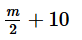•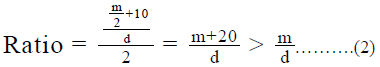3. Set-III: {90, 110, 130, 150}
• ​​{50, 60, 70, 80}→ Multiplying all the terms of the set by 2, results in standard deviation of 2d and mean of 2m. The set becomes = { 100, 120, 140, 160}
• Subtracting 10 from all the terms of the set {100, 120, 140, 160} does not change its standard deviation but decreases the mean by 10. The set becomes = {90, 110, 130, 150}
• Hence, the set {90, 110, 130, 150} has a standard deviation of 2d and mean of 2m – 10
•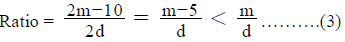4. Comparing (1), (2) and (3), we can arrange the sets in the ratio of mean to standard deviation as
a. III < I < II

QUESTION: 9

Set P has n integers. What is the standard deviation of Set P?
(1) The range of Set P is equal to zero
(2) The mean of Set P is equal to the median of Set P

Solution:

Step 1 & 2: Understand Question and Draw Inference

• Set P→n integers

To Find: Standard Deviation (P)

Step 3 : Analyze Statement 1 independent

(1) The range of Set P is equal to zero

• Range = largest integer – smallest integer
• 0 = largest integer – smallest integer
• largest integer = smallest integer
• This tells us that:
• all the integers of set P are equal OR
• set P has only 1 element

In both the cases, the standard deviation is 0.

Step 4 : Analyze Statement 2 independent

(2) The mean of Set P is equal to the median of Set P

• As the mean of the set is equal to the median of the set, we can say that the set is symmetric about the mean and the median
• However, it does not tell us anything about the other terms of the set. So, we cannot say anything about the standard deviation of the set.

Step 5: Analyze Both Statements Together (if needed)

As we have a unique answer from step- 3, this step is not required.

QUESTION: 10

In triangle ABC (not shown), is the range of the angles of the triangle greater than 90o?
(1) The median angle of triangle ABC is 70o
(2) The difference between the two larger angles of triangle ABC
is 10o

Solution:

Step 1 & 2: Understand Question and Draw Inference

Given: A triangle ABC

• Let the angles of the triangle be x, y, z in increasing order of magnitude.
• We know x + y + z = 180o . . . (1)

To find: Is z – x > 90o ?

Step 3 : Analyze Statement 1 independent

(1) The median angle of triangle ABC is 70o.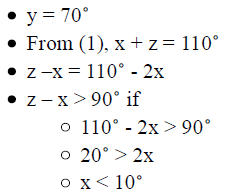But we do not know if x < 10o . So, Statement 1 alone is not sufficient.

Step 4 : Analyze Statement 2 independent

(2) The difference between the two larger angles of triangle ABC is 10o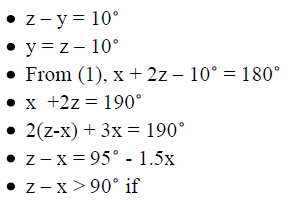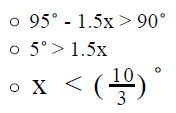But we do not know if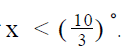So, Statement 2 alone is not sufficient.

Step 5: Analyze Both Statements Together (if needed)

• From Statement 1: y = 70
• From Statement 2: z – y = 10
• By combining these two equations, we’ll get a unique value of z
• From (1), we’ll get a unique value of x

Since we now know the values of x, y and z, we’ll be able to answer the question on the range of the angles.
The two statements together are sufficient to answer the question.

QUESTION: 11

Set P consists of 10 positive integers arranged in order of increasing magnitude. The difference between any two successive
terms of the set is 4. If the two largest terms of the set are removed, what is the decrease in the average(arithmetic mean) of
the set?

Solution:

Given:

• Set P is an arithmetic sequence with common difference(d) = 4 and number of terms(n) = 10
• Let the first term be a.
• So, the other 9 terms = {a+d, a+2d, ……a+9d}
• (a+9d) and (a+8d) are removed

To Find: Decrease in the average of the set after removal of (a+9d) and (a+8d)

Approach:

1. To calculate the decrease in the average of the sequence, we need to calculate the average of the sequences before and after removal of the terms (a+9d) and (a+8d)
2. Calculating Average of the original set P

• As we know all the terms of the set P in terms of a, we can calculate the sum of all the terms in set P using the sum of an arithmetic sequence formula.
• ​Once, we know the sum of an arithmetic sequence P, we can  calculate the average of the arithmetic sequence by dividing the sum by the number of terms(i.e. 10)
3. Calculating Average of set P after removal of (a+9d) and (a+8d)
• The new sum of the arithmetic sequence can be calculated by subtracting the sum of (a+9d) and (a+8d) from the original sum of the arithmetic sequence
• The new average can then be calculated by dividing the new sum by the remaining terms in the sequence(i.e. 8)

Working out:

1. Calculating Average of the original set P
• Sum of the 10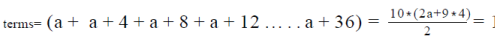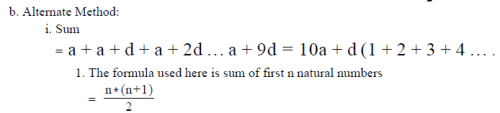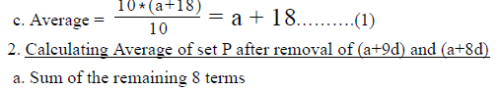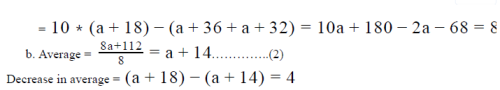Hence, the average decreased by 4 units.

QUESTION: 12

A is the average (arithmetic mean) of the first 7 multiples of 3 and B is the median of the first 3 multiples of positive integer n. If the
value of A2 – B2 is zero, what is the value of n?

Solution:

Given:

• A = Average of {3, 6, 9 . .. 21}
• B = Median of {n, 2n, 3n} where n is a positive integer
• So, B = 2n
• Since n is a positive integer, B must be a positive integer as well
• A2 - B2 =0

To Find: n =?

Approach:

1. To find the value of n, we need to draft an equation in terms of n. From the given information, we can write n= B/2 So, to find n, we need to find the value of B
2. We are given a relation between A2 and B2 . So, if we know the value of A, we can find the value of B
3. We can find the value of A using the given information about A

Working out: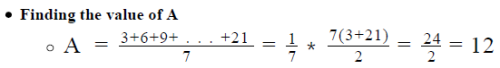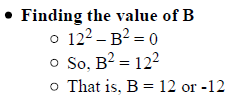• Rejecting the negative value since we’ve inferred above that B is a positive integer
• So, B = 12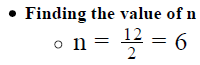Looking at the answer choices, we see that the correct answer is Option D

QUESTION: 13

Set P contains 3 distinct positive integers: A, B and C. Is the average (arithmetic mean) of set P divisible by 3?
(1) The sum of A×104 , B×102 and C is divisible by 9
(2) The product of the range of Set P and the median of Set P is 18.

Solution:

Step 1 & 2: Understand Question and Draw Inference

Given:

• Set P = {A, B, C} where A, B and C are distinct positive integers
• Average of Set P =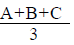To find: Isdivisible by 3?

• If A + B + C divisible by 9?
• Is =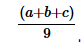an integer?
• Let A = 9m + a, where a is the remainder that A leaves with 9. So a lies between 0 and 8, inclusive
• Similarly let B = 9n + b
• And let C = 9p + c
• So, the question is to find: Is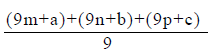an integer?
• Is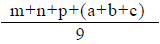an integer?
• Since m + n + p is an integer, the question simplifies to find: is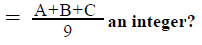Step 3 : Analyze Statement 1 independent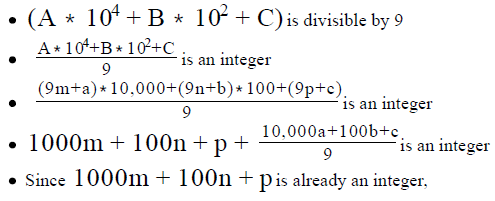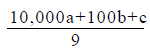• So, the number 10,000a + 100b +c is divisible by 9
• By applying the divisibility rule of 9, we can say that the sum of the digits of the number 10,000a + 100b +c  is divisible by 9
• Since a, b and c are each single-digit integers that lie between 0 and 8, inclusive, the number 10,000a + 100b +c is of the form  a00b0c (that is, a is the ten-thousands digit of this number, b is the hundreds digit and c is the units digit).
• So, the sum of digits of 10,000a +100b+c  (=a00b0c) = a+b+c
• So, a+b+c  is divisible by 9
• So,is an integer

Sufficient.

Step 4 : Analyze Statement 2 independent

• The product of the range of Set P and the median of Set P is 18.
• (Range of Set P)*(Median of Set P) = 18
• Since Set P contains only integers, Range of Set P is an integer
• Since Set P contains an odd number of integers, Median of Set P is equal to the middle integer when A, B and C are arranged in ascending order. Thus, Median of Set P is an integer as well.
• Thus, the product of 2 integers is 18
• The number of ways to express 18 as a product of 2 integers:
• Case 1: 18 = 18*1
• Either Range = 18 and Median = 1
• Not possible – A, B and C are distinct, positive integers. So, minimum value of an integer in Set P is 1. The second integer in Set P cannot also be 1. So, Median cannot be 1
• Or Range = 1 and Median = 18
• Not possible – A, B and C are distinct integers. So, the minimum possible value of Range is 2 (happens if A, B and C are consecutive integers)
• Case 2: 18 = 9*2
• Either Range = 9 and Median = 2
• This means, Set P = {1, 2, 10}
• A + B + C = 1 + 2 +10 = 13, which is not divisible by 9.
• So, the answer to the question is NO
• Or Range = 2 and Median = 9
• This means, Set P = {8, 9, 10}
• A + B + C = 8 + 9 + 10 = 27, which is divisible by 9
• So, the answer to the question is YES
• Case 3: 18 = 6*3
• Since we’ve already seen that Case 2 leads to conflicting answers, we need not analyze Case 3.

Thus, Statement 2 is not sufficient to provide a unique answer to the posed question.

Step 5: Analyze Both Statements Together (if needed)

Since we’ve already arrived at a unique answer in Step 3, this step is not required.

QUESTION: 14

A set consists of n distinct integers arranged in the order of increasing magnitude. Is the median of the n integers equal to the
arithmetic mean of the n integers?
(1) The sum of any 3 successive integers of the set is divisible by 3
(2) The difference between any 2 successive integers of the set is 4

Solution:

Step 1 & 2: Understand Question and Draw Inference

Given: A set of n distinct integers, arranged in the order of increasing magnitude
To find: Is Median = Mean?
The median is equal to the mean if:

• Either the given sequence is an Arithmetic progression
• Or, It’s not an Arithmetic Progression but symmetric about a number.
• Like the set, {1, 2, 4, 6, 7}. This set is {4 – 3, 4 – 2, 4, 4 + 2, 4 + 3}, i.e. it is symmetric about 4.So, the sum of the terms = 4*5 And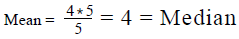Step 3 : Analyze Statement 1 independent

(1) The sum of any 3 successive integers of the set is divisible by 3

• Statement 1 is fulfilled by more than one cases:
• Case 1: The terms of the set are in arithmetic progression
• Example: 1, 2, 3, 4, 5
• In this case, as discussed in Step 1 and 2, Median = Mean (= 3 in the Example above)
• Case 2: The terms of the set are not in arithmetic progression
• For example, a set of the form: {3k + 0, 3k + l, 3k + 5, 3k + 12, 3k + 16} where k is an integer
• In this set,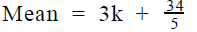• But Median = 3k + 5
• So, Median ≠ Mean
• Thus, Statement 1 doesn’t give us a unique answer to the asked question. So, this statement is not sufficient

Step 4 : Analyze Statement 2 independent

(2) The difference between any 2 successive integers of the set is 4

• Note that we are given that the integers are arranged in ascending order.
• So, combining this fact with Statement 2, we can write that the numbers are of the form: {m, m + 4, m + 8, m + 12, . . . , m + (n-1)*4}
• Thus, the given sequence is an Arithmetic Progression.
• Therefore, the median of the sequence will definitely be equal to the mean of the sequence.

So Statement 2 is sufficient.

Step 5: Analyze Both Statements Together (if needed)

Since we’ve already arrived at a unique answer in Step 4, this step is not required

QUESTION: 15

Set A consists of 15 positive integers. Is the mean of set A equal to the median of set A?
(1) The integers in set A, when arranged in the order of increasing magnitude, are not evenly spaced
(2) If an integer x, which is equal to the mean of set A, is added to the set, the median of the set does not
change

Solution:

Step 1 & 2: Understand Question and Draw Inference

• Set A has 15 positive integers

To Find: Is Mean (A) = Median (A)

Step 3 : Analyze Statement 1 independent

1. The integers in set A, when arranged in the order of increasing magnitude, are not evenly spaced
• For an evenly spaced set, mean = median
• However, for a non-evenly spaced out, mean may or may not be equal to median. For example:
• {1, 2, 5, 8, 9}. Here mean = median = 5
• {1, 3, 6, 8, 22}. Here mean = 8 but median = 6

Step 4 : Analyze Statement 2 independent

2. If an integer x, which is equal to the mean of set A, is added to the set, the median of the set does not change

• When set A consists of 15 terms. Arranging them in ascending order, we have,
• Median = 8 term = let’s say y
• When set A consists of 16 terms, i.e. after the addition of a new term
• Median = Average of 8 and the 9 term = y(as the median did not change)
• 8th term + 9th term = 2y
• Now, let’s observe the position of the term y in the new set A
• If x < y
• Then y would become the 9 term of the new set A
• If x = y
• Then the 8th term and the 9th term both will be equal to y
• If x > y
• Then y would remain the 8 term of the new set A
• So, even after adding of the new term x, y (i.e. the 8 term of the original set A) would either be the 8 term or the 9 term in the new set A
• Hence, from the equation 8 term + 9 term = 2y, we can say that Either(8 term or 9 term) = y
• So, 8 term = 9 term = y
• For the above to hold true, following cases are possible:
• Case-I: mean = median = x .
• This is true for the case, when x = y
• If the same number is added, the mean and the median would not change
• Case-II: When median ≠ mean
• In this case the median ≠ mean and the median value is repeated more than once, such that addition of a new number results in the median being the average of the two repeated median values. For example: Set consisting of {3, 3, 3, 9, 9, 9, 9, 9, 9}. Mean = 7 and
median = 9
If 7 is added, Set = {3, 3, 3, 7, 9, 9, 9, 9, 9, 9}. Mean = 7 and
median = (9+9 ) /2 = 9
• So, the mean = median or the mean ≠ median Insufficient to answer

Step 5: Analyze Both Statements Together (if needed)

1. Set A is not evenly spaced
2. If an integer x, which is equal to the mean of set A, is added to the set, the median of the set does not change

Combining the two statements does not give us any extra information to answer the question.

QUESTION: 16

A merchant sold 32 antique items for \$28800. If his profit margin was 20%, then his average cost per antique item was

Solution:

Given:

• Total Selling Price for 32 items = \$28800
• Profit margin = 20%

To Find: Average cost per item

Approach:

1. Average cost per item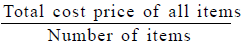• We know that the number of items is 32
• So, to answer the question, we need to know the total cost price of all items

2. We’re given the total selling price of all items as well as the profit margin. Using these 2 pieces of information together, we can find the total cost price of all 32 items

Working out: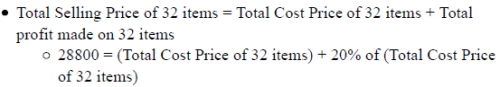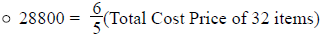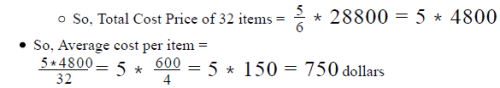Looking at the answer choices, we see that the correct answer is Option B

QUESTION: 17

List A contains 16 distinct odd integers and 9 distinct even integers such that the average (arithmetic mean) of List A is 13.84. If each odd integer is doubled in magnitude, what is the new average (arithmetic mean) of List A?
(1) The average (arithmetic mean) of the even integers in List A is 10
(2) Before each odd integer is doubled in magnitude, the smallest odd integer in List A is 1 and the largest odd integer in List A is
31.

Solution:

Step 1 & 2: Understand Question and Draw Inference

• List A has 16 distinct odd integers and 9 distinct even integers
• Total number of integers in List A = 16 + 9 = 25
• Average of List A = 13.84
• Sum of all integers in List A = 13.84 * 25 = 346
• Let the odd integers in List A be D1 , D2 , D3 . . .D16 Let the sum of these integers be D
• Let the Even integers be E , E , E . . . E . Let the sum of these
• integers be E.
• D + E = 346 . . . (1)
• When each odd number is doubled, the new sum of the odd numbers = 2D.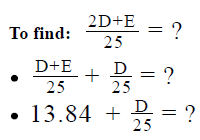So, in order to answer this question, we need to know the value of D.

Step 3 : Analyze Statement 1 independent

(1) The average (arithmetic mean) of the even integers in List A is 10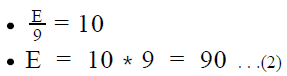Putting (2) in (1), we get the value of D.
So, Statement 1 alone is sufficient.

Step 4 : Analyze Statement 2 independent

(2) Before each odd integer is doubled in magnitude, the smallest odd integer in
List A is 1 and the largest odd integer in List A is 31

• D1 = 1
• D16 = 31
• Number of odd integers between 1 and 31, inclusive, is 16.
• This means, the 16 odd integers in List A are consecutive odd integers starting from 1 and ending at 31.
• Since we now know the values of the odd integers in List A, we can find their sum D.

So, Statement 2 alone is sufficient.

Step 5: Analyze Both Statements Together (if needed)

Since we get a unique answer in each of Steps 3 and 4, this step is not required

QUESTION: 18

While debugging a piece of software, an engineer records the number of bugs he finds each day. If the number of bugs found by the engineer reduces by x with each passing day, what is the standard deviation of the number of bugs found by the engineer during the last 7 days?
(1) The difference between the maximum number of bugs and the minimum number of bugs found by the engineer during the last 7
days is 24.
(2) The average (arithmetic mean) number of bugs found by the engineer during the last 7 days is 24

Solution:

Step 1 & 2: Understand Question and Draw Inference

• Let the number of bugs the engineer found on day 1 = a
• Number of bugs he found on day 2 = a-x
• Number of bugs he found on day 3 = a- x- x = a - 2x
• Number of bugs he found on day n = a - (n-1)x
• Thus the number of bugs found by the engineer is a decreasing Arithmetic Progression.

To Find: standard deviation of the number of bugs found during the last 7 days

• To find the standard deviation of a set, we need to know the following:
• Number of data points in the set
• We are given the number of days = 7
• Distance of each data point of the set from the mean of the set
• We are given the difference between the bugs found on consecutive days = x
• If we know the value of x, we can find the distance of each data point from its mean.
• So, we need to know the value of x to calculate the standard deviation

Step 3 : Analyze Statement 1 independent

• The difference between the maximum number of bugs and the minimum number of bugs found by the engineer during the last 7 days is 24.
• Maximum number of bugs found = (Number of bugs found on day 1) = a
• Minimum number of bugs found = (Number of bugs found on day 7) = a – 6x
• a - (a - 6x) = 24
• x = 6

Step 4 : Analyze Statement 2 independent

2. The average (arithmetic mean) number of bugs found by the engineer during the last 7 days is 24

• Total number of bugs found in during the last 7 days = a + (a –x) +(a-2x) +……(a-6x) = 7a –21x
• Average number of bugs found =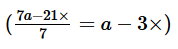• a - 3x = 24

1 equation 2 variablesà cannot find a unique value of x.

Step 5: Analyze Both Statements Together (if needed)

Since, we have a unique answer from step- 3, this step is not required.

QUESTION: 19

A group of 4 boys and 5 girls take a test. What is the average (arithmetic mean) score of the group in the test?

1. The average score of the boys is 23 points while the average score of the girls is 20 points
2. If one of the girls had scored 6 points more, the average score of the group would have been 22
Solution:

Step 1 & 2: Understand Question and Draw Inference

Given: 4 boys and 5 girls take a test
To find: The Average score of the group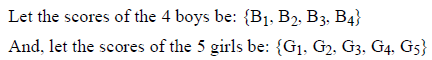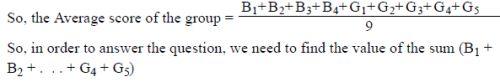Step 3 : Analyze Statement 1 independent

Statement 1 says that ‘The average score of the boys is 23 points while the average score of the girls is 20 points’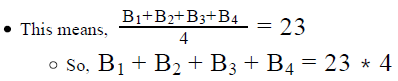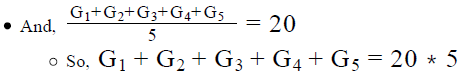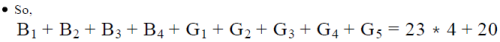• Therefore, we will be able to find the value of the Average Score of the group
• Statement 1 is sufficient to answer the question

Step 4 : Analyze Statement 2 independent

Statement 2 says that ‘If one of the girls had scored 6 points more, the average score of the group would have been 22’

Let the girl who scored 6 points be the first girl (in the list of scores). So, her new score = G + 6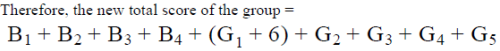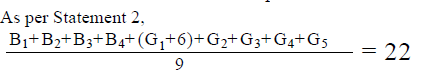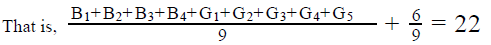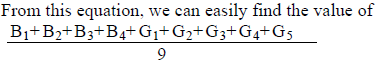So, Statement 2 is sufficient to answer the question

Step 5: Analyze Both Statements Together (if needed)

Since we’ve already arrived at a unique answer in each of Steps 3 and 4, this step is not required

QUESTION: 20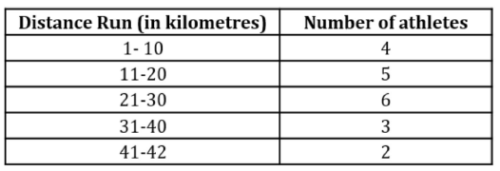The table above shows the distribution of the distance, rounded to the nearest integer, run by 20 athletes in a marathon. Which of the
following cannot be the approximate average (arithmetic mean) distance run (in kilometres) by the athletes in the marathon?

Solution:

Given:

• Number of athletes = 20
• Table showing range of distances, rounded to the nearest integers, run by the athletes

To Find: The option that cannot be the average distance run by the athl

Approach:

1. We need to look for a value that is outside the range of the average distance run by the 20 athletes.
2. We know that the average distance would lie between the minimum possible average distance ran by the athletes and maximum possible average distance ran by the athletes
• So, for finding the range of the distances run, we need to find the maximum and minimum average distances run by the athletes.
• Once we know the maximum and minimum distance run, we can find the range of average distance run by dividing by the number of athletes.
3. Maximum Average Distance
• The average distance ran by the athletes will be maximum when each athlete runs the maximum distance in a given range
• For example: In the range 1- 10 kilometers, each athlete should run 10 kilometers
• Maximum distance run = Sum of (maximum distance run in the range * number of athletes in the range)
• Maximum Average distance run =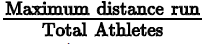Working out: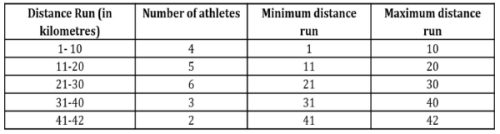1. Minimum distance run = (4*1) + (5*11) +(6*21) + (3*31) +(2*41) = 4 + 55 +126 +93 +82 = 360
• Average of minimum distance run =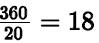2. Maximum distance run = (4 * 10) + ( 5 *20) +(6*30) + (3*40) +(2*42) = 40 + 100 + 180 +120 + 84 = 524 kilometres
• a. Average of maximum distance run =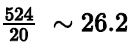3. Hence the range of average distance run by the athletes can be from 18 to 26.2
4. The only option that is outside the range is option E, 28 kilometresUse Code STAYHOME200 and get INR 200 additional OFF Use Coupon Code

Track your progress, build streaks, highlight & save important lessons and more!

Similar ContentRelated tests